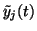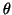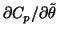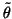Next: Computational Complexity Up: Update Rules Previous: Update Rules for Posterior

## Avoiding Problems Originating from Approximations

When constructing a learning algorithm which is based on approximations of the cost function, it is important to make sure that learning does not drive the network into areas of the parameter space where the approximations are no longer valid.

The approximations in (34) and (35) are based on a roughly quadratic or linear behaviour of the nonlinearities. This assumption is quite good if the posterior varianceof the inputs to the hidden neurons is not very large.

Since the approximations take into account only local behaviour of the nonlinearities gj and MLP networks typically have multimodal posterior distributions, there must be areas of the parameter space where the second order derivative of the posterior probability with respect to one of the parametersis positive. This means thatis negative which in turn means that it appears that the cost function can be made arbitrarily small by lettinggrow.

It is easy to see that the problem is due to the local estimate of gsince the logarithm of the posterior eventually has to go to negative infinity. The derivativewill thus be positive for large, but the local estimate of gj fails to account for this.

In order to discourage the network from adapting itself into areas of parameter space where the problems might occur and to deal with the problem if it nevertheless occurred, the terms in (34) which have negative contribution towill be neglected in the computation of the gradients. As this can only make the estimate ofin (41) smaller, this leads, in general, to increasing the accuracy of the approximations in (34) and (35).Next: Computational Complexity Up: Update Rules Previous: Update Rules for Posterior
Harri Lappalainen
2000-03-03